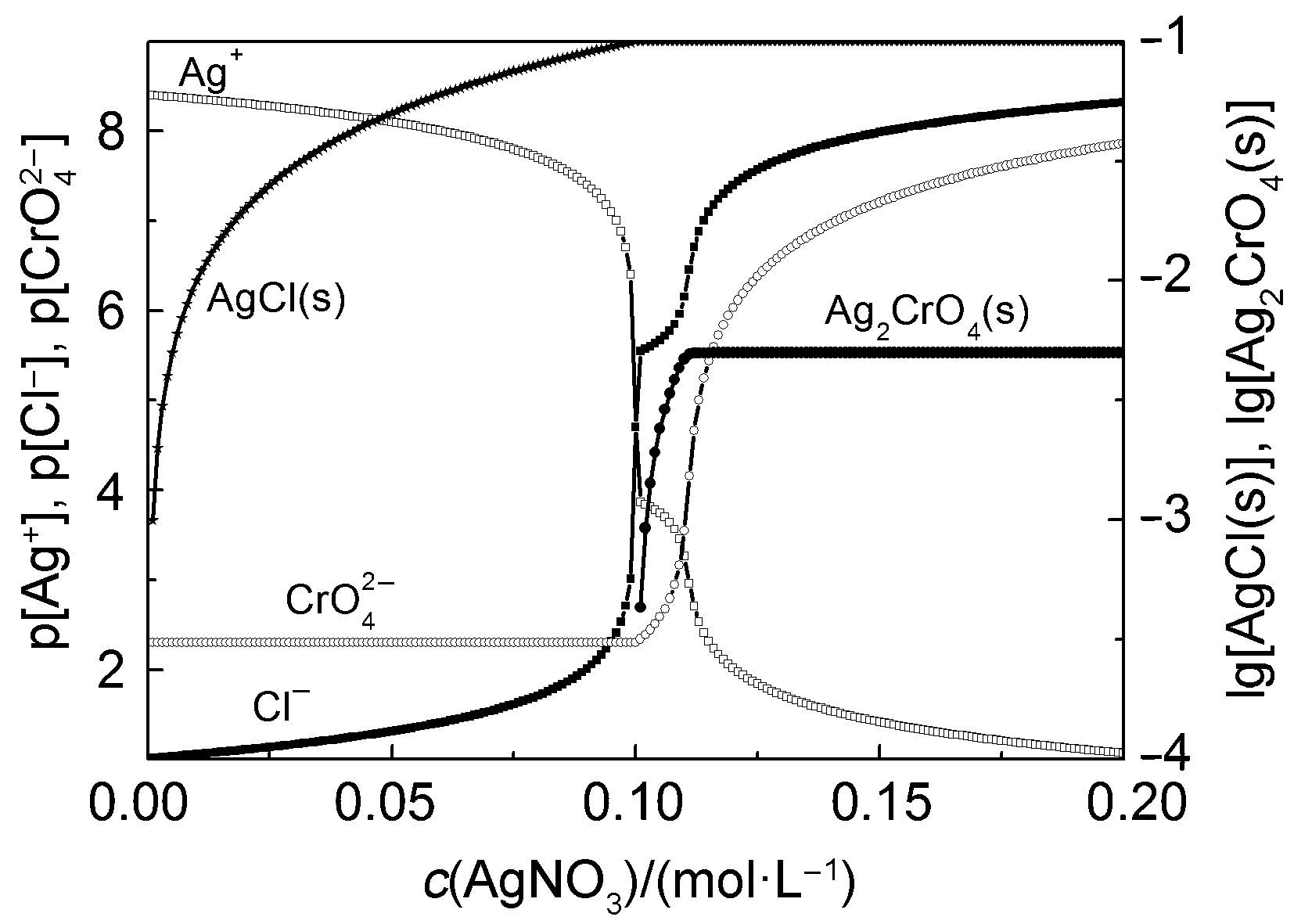## Application of Computer Simulation in Theoretical and Laboratory Teaching of Chemistry,, ,

 基金资助: 广东省高等教育教学改革项目（本科类）.  45000-18822503中山大学教学改革研究资助项目.  45000-16300004

 Fund supported: 广东省高等教育教学改革项目（本科类）.  45000-18822503中山大学教学改革研究资助项目.  45000-16300004Abstract

With the help of PHREEQC program,we introduced the application of computer simulation in theoretical and laboratory teaching of chemistry.Accordingly,reaction models for the chemical process of acid-base neutralization,complexation reaction,precipitation-dissolution equilibrium,and chemical kinetics were established.These models can greatly help the students understand chemistry,improve the teaching quality,enrich modern teaching methodology,develop students' creativity,and establish a scientific and efficient research method.

Keywords： Multimediateaching ; Chemical simulation ; PHREEQCprogram ; Modernteachingmethod

KANG Ming-Liang, HAN Dong-Mei, GEWIRTZ Océane. Application of Computer Simulation in Theoretical and Laboratory Teaching of Chemistry. University Chemistry[J], 2016, 31(10): 23-28 doi:10.3866/PKU.DXHX201602012

## 3 教学组织模式

### 图1### 4.2 络合反应

EDTA全称为乙二胺四乙酸(H4Y)，是一种良好的络合剂，它有6个配位原子，形成的络合物叫做螯合物。EDTA滴定法是化学分析中常用的分析方法，有直接滴定、间接滴定、返滴定和置换滴定，可用于水的总硬度、溶液中多种金属离子含量的测定。

${{H}_{6}}{{Y}^{2+}}\left( aq \right)={{H}^{+}}\left( aq \right)+{{H}_{5}}{{Y}^{+}}\left( aq \right)\quad {{K}_{a1}}={{10}^{-0.9}}$

${{H}_{5}}{{Y}^{+}}\left( aq \right)={{H}^{+}}\left( aq \right)+{{H}_{4}}{{Y}^{+}}\left( aq \right)\quad {{K}_{a2}}={{10}^{-1.6}}$

${{H}_{4}}Y\left( aq \right)={{H}^{+}}\left( aq \right)+{{H}_{3}}{{Y}^{-}}\left( aq \right)\quad {{K}_{a3}}={{10}^{-2.0}}$

${{H}_{3}}{{Y}^{-}}\left( aq \right)={{H}^{+}}\left( aq \right)+{{H}_{2}}{{Y}^{2-}}\left( aq \right)\quad {{K}_{a4}}={{10}^{-2.67}}$

${{H}_{2}}{{Y}^{2-}}\left( aq \right)={{H}^{+}}\left( aq \right)+H{{Y}^{3-}}\left( aq \right)\quad {{K}_{a5}}={{10}^{-6.16}}$

$H{{Y}^{3-}}\left( aq \right)={{H}^{+}}\left( aq \right)+{{H}_{5}}{{Y}^{4-}}\left( aq \right)\quad {{K}_{a6}}={{10}^{-10.26}}$

### 图2PHREEQC允许用户根据自己的数据对使用的热力学数据库进行添加更新，因此我们将上述反应式(1) -式(6)添加至常用的llnl.dat中。 PHREEQC采用WATEQ推广的Debye- Hückel公式 $\lg {{\gamma }_{i}}=-\frac{AZ_{i}^{2}\sqrt{I}}{1+B{{a}_{i}}\sqrt{I}}+{{b}_{i}}I$ 计算溶液中各物种的活度系数γi，公式中参数A B 在定温下是常数，Z i为离子电荷数，I 为溶液的离子强度，ai为离子i 的特征参数，ai bi 也称为Debye-Hückel参数；对于未给定Debye-Hückel参数的离子，PHREEQC采用Davies公式 $\lg {{\gamma }_{i}}=-AZ_{i}^{2}\left( \frac{\sqrt{I}}{1+\sqrt{I}}-0.3I \right)$ 计算离子活度系数γi；对于不带电粒子，则使用公式lgγi = 0.1I 计算活度系数。图3是使用PHREEQC计算的EDTA形态分布随pH的变化图。由模拟结果来看，两个相邻形态的优势区分隔线并不是pH = 0.9、 1.6、2.0、2.67、6.16和10.26，而是更小的pH。通过图2图3的直观比较，可以使学生更容易理解并掌握化学平衡常数及活度的概念。

### 图3EDTA极易与金属离子形成1 : 1的螯合物，与Ca2+和Mg2+形成配合物的稳定常数lgK 分别为10.7和8.7。在pH = 10的NH3-NH4Cl缓冲溶液中，以铬黑T为指示剂，用EDTA标准溶液滴定溶液中的Ca2+、Mg2+，可以测定水的总硬度及钙镁离子含量，这是基础化学的实验教学内容。依据这些实验条件(钙镁离子浓度均设为0.02 mol∙L-1)及热力学参数，PHREEQC可动态模拟整个实验过程(图4)。由于程序可随时改变模拟条件，以考查不同条件下的实验结果，因此计算机模拟能很好地辅助化学实验教学。基础化学中其他常见的络合反应，如NH3滴定AgNO3、AgNO3滴定CN-、Hg(NO3)2滴定Cl-、Cu2+/Fe3+对SCN-的络合竞争反应、或SCN-/C2O42-对Fe3+的竞争络合，也均可利用PHREEQC来建立反应模型，在此不详述。

### 图4pH = 10；Ca2+/Mg2+的浓度为0.02 mol∙L-1

### 图51-UO22+ ；2-UO2OH+ ；3-(UO2)3(OH)5+；4-UO2(OH)2；5-(UO2) 2CO3(OH)3- ；6-pH；7-UO2(OH)3- ；8-UO2(CO3) 22- ；9-UO2(CO3) 34-

### 图6[Cl-] = 0.1 mol∙L-1；[CrO4 2-] = 5 mmol∙L-1

### 图7## 参考文献 原文顺序 文献年度倒序 文中引用次数倒序 被引期刊影响因子

http://wwwbrr.cr.usgs.gov/projects/GWC_coupled/phreeqc/

Singer P. C. ; Stumm W. Science 1970, 167, 1121.

/

 〈〉